# Effectively Running a Harmonic Analysis in SOLIDWORKS Simulation

One of the most powerful capabilities in SOLIDWORKS Simulation Premium is linear dynamics.  It encompasses a subset of dynamic analysis that is common in many industries, and can include the following types of analyses: transient, harmonic, random vibration and shock response spectrum analysis.  The intent of this blog post is to give you a solid footing and procedure to walk through when you are required to run a harmonic analysis.  Let’s first start with a description of exactly what linear dynamics means.

With linear dynamic analysis, the loads are applied with respect to time or frequency, they can be defined in a deterministic nature (periodic or non-periodic) or non-deterministic, which means they are not precise but defined statistically.  Unlike linear static analysis, where only the stiffness, displacement and load are important, with a full linear dynamic system now the damping and mass matrices are included in the equations of motion.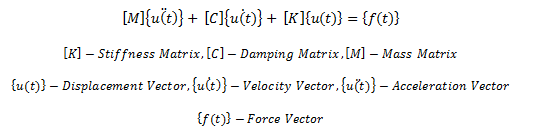The stiffness, damping and mass matrices are fixed with respect to time.  No material nonlinearity can be included, and there can’t be changes in contact, things can either be bonded or free, otherwise the stiffness of the structure would change.  Finally, small deflection theory is required.  If any of those criteria are not met, we would need to move from linear dynamics to non-linear dynamic analysis, which is also available in SOLIDWORKS Simulation Premium.

Harmonic analysis assumes that the applied loads vary as a sine or cosine function.

Example: A reciprocating pump, weighing 150lb, is mounted at the middle of a steel plate of thickness 0.5 in., width 20in., and length 100 in., clamped along two edges. During operation of the pump, the plate is subjected to a harmonic force,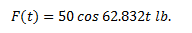Our engineering challenge is to find the amplitude of vibration of the steel plate (Reference: Rao 199).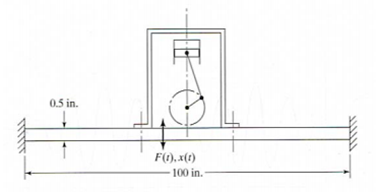The approach I like to follow is to first solve a modal analysis problem and/or a static deflection problem.  For this example we can do both very quickly, but first let’s investigate the assumptions made in the referenced text to solve this problem.  The plate is modeled as a clamped-clamped beam, and the weight is neglected.  The material properties for the beam are assumed to be E=30E6 psi.  Using these assumptions, we can come up with the closed form solution to this problem.

Solution: The bending stiffness of the beam is given by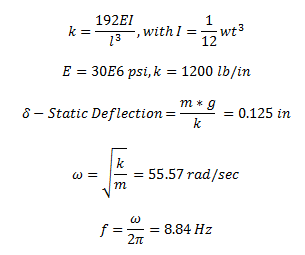The amplitude of the harmonic response is given by the following equation (Eq 3.6 Rao):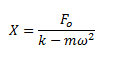With Fo = 50 lb, and m = 150/386.4 lb-sec2/in.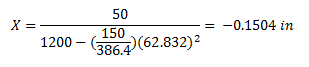The negative sign indicates that the response X(t) of the plate is out of phase with the excitation F(t).

Now let’s walk run this with SOLIDWORKS Simulation.  First we model the plate, and at the center we can put a 1 in3 block.  We customize the materials so the plate has the appropriate modulus with a density of nearly zero (as the mass has been neglected in the referenced text).  By making the block 1 in3 we can input density as 150lbf/in3.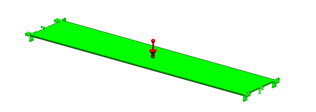Let’s now solve for static deflection and frequency.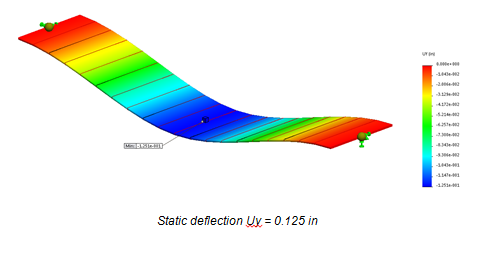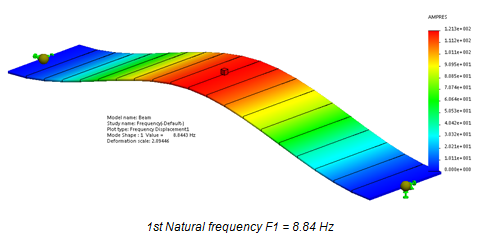Finally, we setup and run our harmonic analysis.  The harmonic force definition gives us the excitation frequency as 62.832 rad/sec, which correlates to 10 Hz.  With linear dynamic studies we are required to run a modal analysis first, and the modes and the mass participation of those modes drive the accuracy of the linear dynamic analysis.  For a case like where we are driving the model in the y-direction you want enough modes to get 90% of the mass in the driving axis.  You can check this when you run your modal analysis by looking at the mass participation table.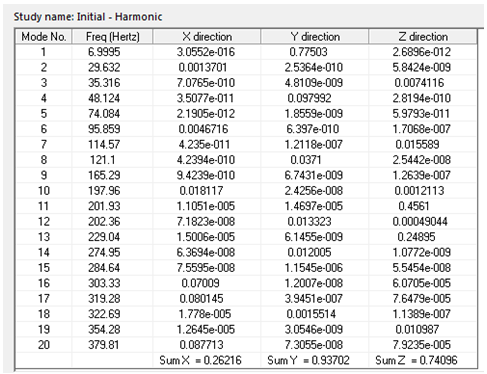So, we have 93% of the mass in the y-axis if we pull 20 modes, more than adequate for this run.  We’ll also need input some damping; normally we use modal damping and a damping ratio.  SOLIDWORKS Simulation also allows for Rayleigh (?-?) damping, or you can enter damping in the material properties.  In this case, since damping isn’t specified in the referenced text we input the smallest possible damping 1E-5 ratio.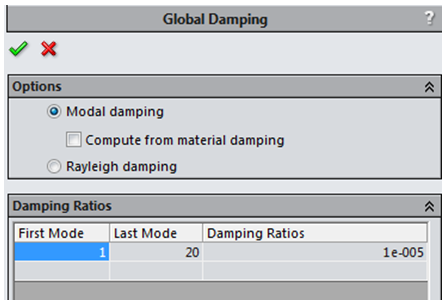The final input is just the force. With harmonic analysis any loads you apply are assumed to be applied harmonically.  In this case we have a 50 lbf load applied on mass. The amplitude of displacement at 10 Hz is then 0.15 in: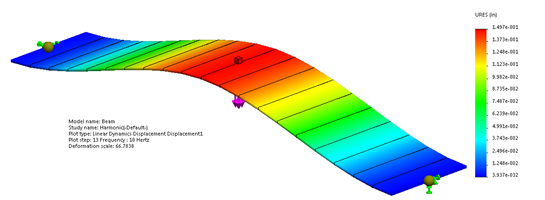So, we can see when the problem is characterized correctly the correlation between closed form and FEA solution is very close.

 Closed Form (Rao) FEA (SOLIDWORKS Simulation) Static Deflection (in) 0.125 0.1251 Resonant Frequency (Hz) 8.845 8.843 Harmonic Response @ 10 Hz (in) 0.1504 0.1497

There are three other types of linear dynamic studies which will be covered in future blogs; linear transient, random vibration and shock response spectrum. The procedures to effectively run those are similar to harmonic, but they all have their little intricacies. If you have any questions reach out to the technical resources at your resellers#### Omar Zohni

Senior Territory Manager on the SOLIDWORKS Simulation Team